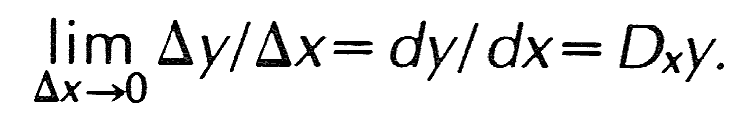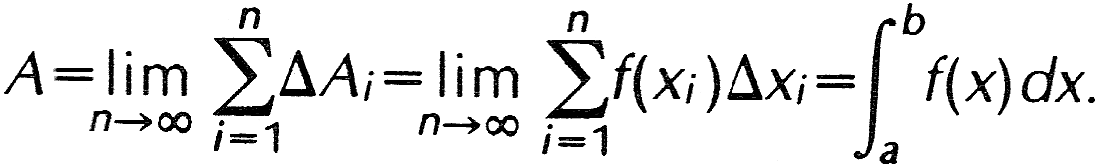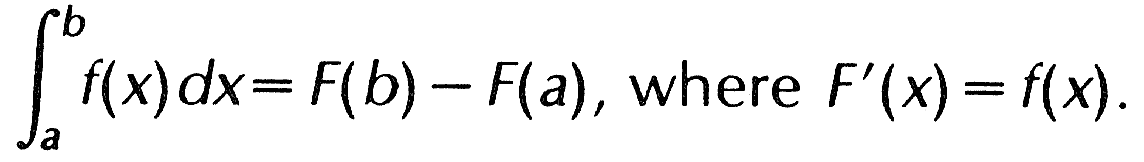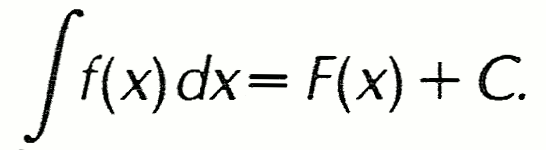تخطي للمحتوى الرئيسي تخطي لصندوق البحث
Summary Article: calculus
From The Columbia Encyclopedia

branch of mathematics that studies continuously changing quantities. The calculus is characterized by the use of infinite processes, involving passage to a limit—the notion of tending toward, or approaching, an ultimate value. The English physicist Isaac Newton and the German mathematician G. W. Leibniz, working independently, developed the calculus during the 17th cent. The calculus and its basic tools of differentiation and integration serve as the foundation for the larger branch of mathematics known as analysis. The methods of calculus are essential to modern physics and to most other branches of modern science and engineering.

The Differential Calculus

The differential calculus arises from the study of the limit of a quotient, Δyx, as the denominator Δx approaches zero, where x and y are variables. y may be expressed as some function of x, or f(x), and Δy and Δx represent corresponding increments, or changes, in y and x. The limit of Δyx is called the derivative of y with respect to x and is indicated by dy/dx or Dxy:The symbols dy and dx are called differentials (they are single symbols, not products), and the process of finding the derivative of y=f(x) is called differentiation. The derivative dy/dx=df(x)/dx is also denoted by y′, or f′(x). The derivative f′(x) is itself a function of x and may be differentiated, the result being termed the second derivative of y with respect to x and denoted by y″, f″(x), or d2y/dx2. This process can be continued to yield a third derivative, a fourth derivative, and so on. In practice formulas have been developed for finding the derivatives of all commonly encountered functions. For example, if y=xn, then y′=nxn − 1, and if y=sin x, then y′=cos x (see trigonometry). In general, the derivative of y with respect to x expresses the rate of change in y for a change in x. In physical applications the independent variable (here x) is frequently time; e.g., if s=f(t) expresses the relationship between distance traveled, s, and time elapsed, t, then s′=f′(t) represents the rate of change of distance with time, i.e., the speed, or velocity.

Everyday calculations of velocity usually divide the distance traveled by the total time elapsed, yielding the average velocity. The derivative f′(t)=ds/dt, however, gives the velocity for any particular value of t, i.e., the instantaneous velocity. Geometrically, the derivative is interpreted as the slope of the line tangent to a curve at a point. If y=f(x) is a real-valued function of a real variable, the ratio Δyx=(y2 − y1)/(x2 − x1) represents the slope of a straight line through the two points P (x1,y1) and Q (x2,y2) on the graph of the function. If P is taken closer to Q, then x1 will approach x2 and Δx will approach zero. In the limit where Δx approaches zero, the ratio becomes the derivative dy/dx=f′(x) and represents the slope of a line that touches the curve at the single point Q, i.e., the tangent line. This property of the derivative yields many applications for the calculus, e.g., in the design of optical mirrors and lenses and the determination of projectile paths.

The Integral Calculus

The second important kind of limit encountered in the calculus is the limit of a sum of elements when the number of such elements increases without bound while the size of the elements diminishes. For example, consider the problem of determining the area under a given curve y=f(x) between two values of x, say a and b. Let the interval between a and b be divided into n subintervals, from a=x0 through x1, x2, x3, … xi − 1, x+i, … , up to xn=b. The width of a given subinterval is equal to the difference between the adjacent values of x, or Δxi=xi − xi − 1, where i designates the typical, or ith, subinterval. On each Δxi a rectangle can be formed of width Δxi, height yi=f(xi) (the value of the function corresponding to the value of x on the right-hand side of the subinterval), and area ΔAi=f(xixi. In some cases, the rectangle may extend above the curve, while in other cases it may fail to include some of the area under the curve; however, if the areas of all these rectangles are added together, the sum will be an approximation of the area under the curve.

This approximation can be improved by increasing n, the number of subintervals, thus decreasing the widths of the Δx's and the amounts by which the ΔA's exceed or fall short of the actual area under the curve. In the limit where n approaches infinity (and the largest Δx approaches zero), the sum is equal to the area under the curve:The last expression on the right is called the integral of f(x), and f(x) itself is called the integrand. This method of finding the limit of a sum can be used to determine the lengths of curves, the areas bounded by curves, and the volumes of solids bounded by curved surfaces, and to solve other similar problems.

An entirely different consideration of the problem of finding the area under a curve leads to a means of evaluating the integral. It can be shown that if F(x) is a function whose derivative is f(x), then the area under the graph of y=f(x) between a and b is equal to F(b) − F(a). This connection between the integral and the derivative is known as the Fundamental Theorem of the Calculus. Stated in symbols:The function F(x), which is equal to the integral of f(x), is sometimes called an antiderivative of f(x), while the process of finding F(x) from f(x) is called integration or antidifferentiation. The branch of calculus concerned with both the integral as the limit of a sum and the integral as the antiderivative of a function is known as the integral calculus. The type of integral just discussed, in which the limits of integration, a and b, are specified, is called a definite integral. If no limits are specified, the expression is an indefinite integral. In such a case, the function F(x) resulting from integration is determined only to within the addition of an arbitrary constant C, since in computing the derivative any constant terms having derivatives equal to zero are lost; the expression for the indefinite integral of f(x) isThe value of the constant C must be determined from various boundary conditions surrounding the particular problem in which the integral occurs. The calculus has been developed to treat not only functions of a single variable, e.g., x or t, but also functions of several variables. For example, if z=f(x,y) is a function of two independent variables, x and y, then two different derivatives can be determined, one with respect to each of the independent variables. These are denoted by ∂z/∂x and ∂z/∂y or by Dxz and Dyz. Three different second derivatives are possible, ∂2z/∂x2, ∂2z/∂y2, and ∂2z/∂xy=∂2z/∂yx. Such derivatives are called partial derivatives. In any partial differentiation all independent variables other than the one being considered are treated as constants.

Bibliography
• See ; , Introduction to Calculus and Analysis, Vol. I (1965);.
• , Calculus: An Intuitive and Physical Approach (2 vol., 1967);.
• ; , Calculus and Analytic Geometry (7th ed. 2 vol., 1988).The Columbia Encyclopedia, © Columbia University Press 2018

### مقالات كريدو ذات الصلة

##### Full text Article Calculus
Bloomsbury Guide to Human Thought

The word calculus is originally Latin for a small stone, referring to those used to perform calculations on an abacus or similar tool. Today...

##### Full text Article calculus
Dictionary of World Philosophy

A system of symbols by means of which inferences can be made by manipulating its symbols in accordance with its rules. A calculus consists of...

##### Full text Article calculus
The Macmillan Encyclopedia

The mathematical techniques, developed by Newton and Leibniz , based on the concept of infinitely small changes in continuously varying...

مشاهدة المزيد من كريدو